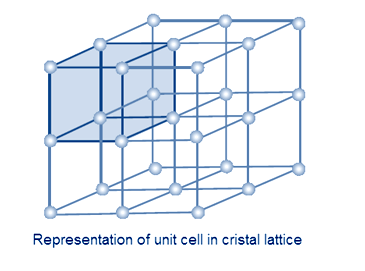# Question #96797

Nov 20, 2016

There are four sodium ions in a unit cell of sodium chloride.

#### Explanation:

The unit cell

A unit cell is the smallest group of particles that constitutes the repeating pattern in a crystal.

Each unit cell is surrounded by other unit cells in all directions to make up a crystal lattice.The $\text{NaCl}$ unit cell

Here is a diagram of the NaCl unit cell.It is called a face centred cubic cell because the large chloride ions (green) are at the faces of a cube.

The smaller sodium ions (grey) fill in the holes around the edges of the cube.

Here is an expanded view of the unit cell.You can see that there is also a sodium ion in the centre of the cube, and it does not belong to any other unit cell.

Number of ${\text{Na}}^{+}$ ions in unit cell

Four other cubes share each edge of the cube, so there is ¼ of a sodium ion at each edge.

There are four edges at the top, four at the bottom, and four at the sides.

$\text{Number of Na"^+ "ions" = 12 color(red)(cancel(color(black)("edges"))) × (1/4 color(white)(l)"ion")/(1 color(red)(cancel(color(black)("edge")))) + 1 color(red)(cancel(color(black)("centre")))× "1 ion"/(1 color(red)(cancel(color(black)("centre")))) = "3 ions + 1 ion" = "4 ions}$

There are four sodium ions in a unit cell of sodium chloride.